A Source Book in Mathematics, 1200-1800

Contents:
Author: Isaac Newton  | Date: 1860

Show Summary
Mathematics

8 NEWTON.

# Cubic Curves

### SECTION I. THE ORDER OF LINES

Geometrical lines1 are best divided into orders, according to the dimensions of the equation expressing the relation between absciss and ordinate, or, which is the same thing, according to the number of points in which they can be cut by a straight line. So that a line of the first order will be a straight line; those of the second or quadratic order will be conic sections and the circle; and those of the third or cubic order will be the cubic Parabola, the Neilian Parabola, the Cissoid of the ancients, and others we are about to describe. A curve of the first genus (since straight lines are not to be reckoned among curves) is the same as a line of the second order, and a curve of the second genus is the same as a line of the third order.2 And a line of the infinite order is one which a straight line may cut in an infinite number of points, such as the spiral, cycloid, quadratrix, and every line generated by the infinitely continued rotations of a radius.

### SECTION II. THE PROPERTIES OF CONIC SECTIONS ARE ANALOGOUS TO THOSE OF CURVES OF HIGHER ORDERS

The chief properties of conic sections have been much treated of by geometers, and the properties of curves of the second and higher genera are very similar to them, as will be shown in the following enumeration of their principal properties:

1. Of Curves of the Second Genus, Their Ordinates, Diameters, Vertices, Centres, and Axes. If parallel straight lines terminated by the curve be drawn in a conic section, the straight line bisecting two of them will bisect all the others, and is called a diameter, and the bisected fines are called ordinates to the diameter, and the intersection of all the diameters is the centre; the intersection of the diameter with the curve is called the vertex; that diameter, whose ordinates are rectangular to it, being called the axis. In like manner, in curves of the second genus, if any two parallel straight lines are drawn, meeting the curve in three points; the straight line which cuts these parallel lines so that the sum Of the two segments meeting the curve on one side of the secant, will cut in the same ratio all fines parallel to these, provided they also meet the curve in three points; that is, so that the sum of the two parts on one side of tim secant, shall equal the third part on the other side. These three parts, thus equal, may be called ordinates, and the secant or cutting line to which the ordinates arc applied, the diameter; the intersection of diameter and curve the vertex; and the intersection of two diameters, the centre. The diameter having rectangular ordinates, if any exist, may also be called aa axis; and where all the diameters meet in a point, that point will be the general centre.

2. Of Asymptotes and Their Properties. A hyperbola of the first genus will have two asymptotes; that of the second genus will have three; of the third, four, and no more; and so on for the rest. And as the segments of any straight line intercepted between the conic hyperbola and its asymptotes on each side are equal, so in hyperbolas of the curve and its three asymptotes in three points, the sum of those two segments of the secant, which are drawn from any two asymptotes on the same side to two points of the curve, will be equal to the third part, which is drawn from the third asymptote on the contrary side, to the third point in the curve.3

3. Of Latera Recta and Transversa. And in like manner, as in the nonparabolic conic sections, the square of the ordinate—i.e., the rectangle contained by the ordinates on each side of the diameter4— is to the rectangle of the segments of the diameter of the ellipse and hyperbola, terminated at the vertices; as a certain given line called the latus rectum, to the part of the diameter which lies between the vertices, and is called the latus transversum; so in nonparabolic curves of the second genus, the product of three ordinates, is to the product of the abscisses of the diameter between the ordinates and the three vertices of the curve, in a given ratio; in which ratio, if three lines are taken to three segments of the diameter between the vertices of the curve, each to each, then these three lines may be considered as the latera recta of the curve, and the three segments of the diameter between the vertices as its latera transversa. And as in the conic parabola, which has but one vertex to a diameter, the rectangle under the ordinates, is equal to the rectangle under the absciss between the vertex and ordinates, and a given straight line called the latus rectum: so in curves of the second genus, which have only two vertices to the same diameter, the product of the three ordinates is equal to the product of the two parts of the diameter cut off between the ordinates and the two vertices, and a certain given straight line, which may be called the latus rectum.5

5. Of Hyperbolic and Parabolic Branches, and Their Directions. All infinite branches of curves of the second and higher genus, like those of the first, are either of the hyperbolic or parabolic sort. I define a hyperbolic branch aa one which constantly approaches some asymptote, a parabolic branch to be that which, although infinite, has no asymptote. These branches are easily distinguished by their tangents; for supposing the point of contact to be infinitely distant, the tangent of the hyperbolic branch will coincide with the asymptote, but the tangent of the parabolic branch being at an infinite distance, vanishes, and is not to be found. The asymptote to any branch is, therefore, found by seeking for the tangent to a point in that branch at an infinite distance. The direction of the branch may be found, by determining the position of a straight line parallel to the tangent referred to a point in the curve infinitely distant; for such straight line will have the same direction as the infinite branch itself.

### SECTION III. THE REDUCTION OF ALL CURVES OF THE SECOND GENUS TO FOUR CASES OF EQUATIONS

All lines of the first, third, fifth, seventh, or odd orders, have at least two infinite branches extending in opposite directions; and all lines of the third order have two branches of the same kind, proceeding in opposite directions, towards which no other of their infinite branches proceed (except only the Cartesian parabola).

Case I (Fig. 1). If the branches be hyperbolic, let GAS be their asymptote, and let CBc be any line drawn parallel to it, meeting the curve on each side (if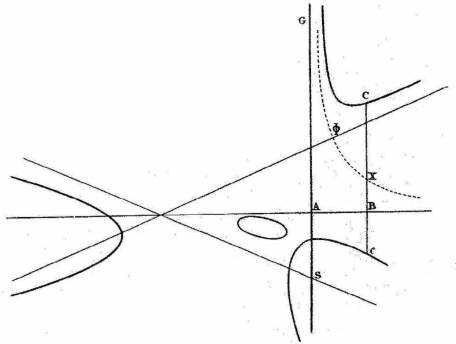Fig. 1

possible); let this line be bisected in X, which will be the locus of a hyperbola, say , one of whose asymptotes is AG. Let its other asymptote be AB, and the equation definining the relation between absciss AB and ordinate BC, if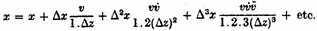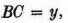will always be of the form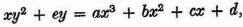where the terms b, c, d, a, e, designate given quantities, affected by their proper signs + or -, of which any may be deficient, so that the figure, by reason of their absence, be not changed to a conic section. It may be, however, that this conic hyperbola coincides with its asymptotes; that is, the point X may fall in the straight line AB; and in that ease the term +ey is deficient.

Case II (Fig. 2). But if the straight line CBc is not bounded by the curve at each end, but only meets the curve in one point, draw AB any straight line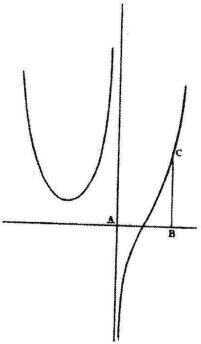Fig. 2

given in position, meeting the asymptote AS in A, and draw another line BC parallel to that asymptote, and meeting the curve in C; then the equation expressing the relation of the ordinate BC and absciss AB always assumes the form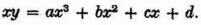Case III (Fig. 3). But if the opposite branches are of the parabolic sort, let the straight line CBc be drawn, if possible, meeting each branch of the curve, and being bisected in B, the locus of B will be a straight line. Let this straight line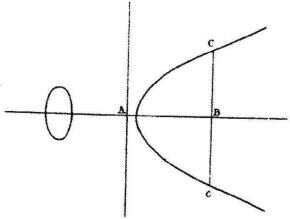Fig. 3

be AB, terminating at any point A, then the equation expressing the relation of ordinate BC and absciss AB always assumes the form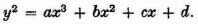Case IV (Fig. 4). But when CBc only meets the curve in one point, and, therefore, cannot be bounded at both ends, let that one point be C; and at the point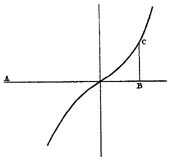Fig. 4

B let CBc meet another straight line given in position, AB, and terminating at any point A, then the equation expressing the relation between ordinate BC and absciss AB always assumes the form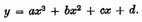The Names of the Curves. In the enumeration of these cases of curves, we shall call that which is included within the angle of the asymptotes in like manner as the hyperbola of the cone, the inscribed hyperbola; that which cuts the asymptotes, and includes within its branches the parts of the asymptotes so cut off, the circumscribed hyperbola; that which, as to one branch, is inscribed, and, as to the other, circumscribed, we shall call the ambigenous hyperbola; that which has branches concave to each other and proceeding towards the same direction, the converging hyperbola; that which has branches convex to each other, and proceeding towards contrary directions, the diverging hyperbola; that which has branches convex to contrary parts and infinite towards contrary sides, the contrary branched hyperbola; that which, with reference to its asymptote, is concave at the vertex, and has diverging branches, the conchoidal hyperbola; that which cuts the asymptote in contrary flexures, having on both sides contrary branches, the serpentine hyperbola; that which intersects its conjugate, the cruciform hyperbola; that which intersects and returns in a loop upon itself, the nodate hyperbola; that which has two branches meeting at an angle of contact, and there stopping, the cusped hyperbola; that which has an infinitely small conjugate oval, i.e., a conjugate point, the punctate hyperbola; that which, from the impossibility of two roots, has neither oval, node, cusp, nor conjugate point, the pure hyperbola.

In the same way we shall speak of parabolas, as converging, diverging, contrary branched, cruciform, nodate, cusped, punctate, and pure.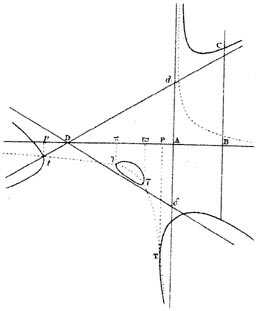Fig 5.

In the case of the first-mentioned equation, if ax3 is positive (Fig. 5), the figure will be a triple hyperbola with six hyperbolic branches progressing to infinity alongside of three asymptotes, no one of which is parallel to another, two alongside of each hyperbola, on contrary sides. And these asymptotes, if the term bx2 is not deficient, will cut one another at three points, making a triangle (Ddδ); but if the term bx2 is deficient, all the points will converge to one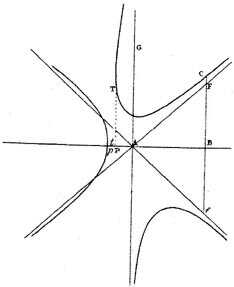Fig 6.

point. In the former ease, take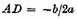and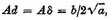join Dd, Dδ; and Ad, Dd, Dδ will be the three asymptotes. In the latter case (Fig. 6) draw any ordinate BC parallel to the principal ordinate AG, and in it produced in each direction take BF, Bf, equal to each other, and in the ratio to AB of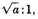join AF, Af; and AG, AF, Af will be the three asymptotes. This hyperbola we call redundant, because it exceeds the conic hyperbola in the number of its hyperbolic branches.

### SECTION IV. THE ENUMERATION OF CURVES. OF THE NINE REDUNDANT HYPERBOLAS, HAVING NO DIAMETER, AND THREE ASYMPTOTES, MARKING A TRIANGLE

When a redundant hyperbola has no diameter (Fig. 5), let the four roots be found of the equation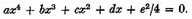Erect the ordinates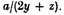pt which are tangent to the curve at the points T, τ, l, t, and by touching give the limits of the curve by which this species is determined. Indeed, if all the roots AP,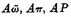(Figs. 5, 7), are real and unequal, and of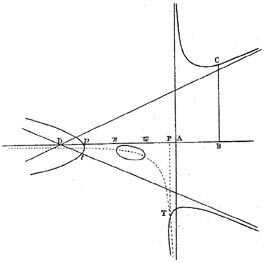Fig. 7

the same sign, the curve consists of three hyperbolas (inscribed, circumscribed, and ambigenous), and of an oval. One hyperbola lies towards D, another towards d, and the third towards δ; and the oval always lies within the triangle Ddδ within the limits τl, in which it is touched by the ordinates πl and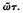This is the 1st species.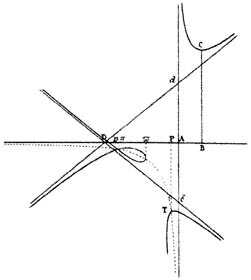Fig. 8

If the two greatest roots Aπ, AP (Fig. 8), or the two least AP,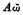(Fig. 9), equal to each other, and all of the same sign, the oval and circumscribed hyperbola will coalesce, their points of contact l and t, or T and τ, coming together, and the branches of the hyperbola, intersecting one another, run on into the oval, making the figure nodate.

This is the 2d species.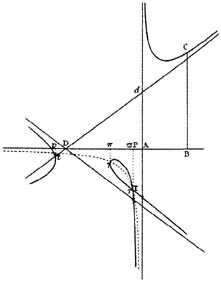Fig. 9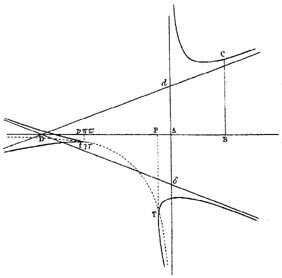Fig. 10

If the three greatest roots AP, Aπ, Aω (Fig. 10), or the three least roots Aπ,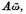AP (Fig. 11), are equal to each other, the node becomes a sharp cusp; because the two branches of the circumscribed hyperbola meet at an angle of contact, and extend no farther.

This is the 3d species.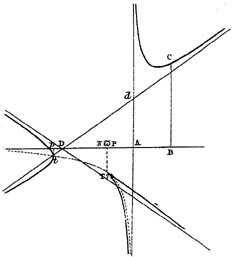Fig. 11

### SECTION V THE GENERATION OF CURVES BY SHADOWS

7

If the shadows of curves caused by a luminous point, be projected on an infinite plane, the shadows of conic sections wilt always be conic sections; those of curves of the second genus will always be curves of t he second genus; those of the third genus will always be curves of the third genus; and so on ad infinitum.

And in the same manner as the circle, projecting its shadow, generates all the conic sections, so the five divergent parabolas, by their shadows, generate all other curves of the second genus. And thus some of the more simple curves of other genera might be found, which would form all curves of the same genus by the projection of their shadows on a plane.

1 Descartes, in his Géométrie, Book II, calls curves that can be expressed by a single equation (which in his case is always an algebraic equation), "courbes géométriques"; see D. E. Smith and M. L. Latham, trans., The Geometry of René Descartes (Open Court, Chicago, London, 1925; Dover, New York, 1954), 48.

2 This division of geometrical curves into curves of genus [genre]1 (conic sections), genus 2 (third- and fourth-degree curves), genus 3 (curves of degree 5 and 6) can also be found in Descartes, on the same page as referred to in note 2; see Selection III.4, note 1.

3 This can be proved with the aid of a diameter. For a full explanation see Talbot, Newton’s "Enumeration," p. 37.

4 It will be remembered that "the rectangle contained by two lines x and y" means the product xy.

5 See Talbot, Newton’s "Enumeration," pp. 38–40 for full documentation.

7 This short paragraph on the genesis curvarum per umbras contains Newton’s theorem that every curve of the third order can be obtained from a divergent parabola by central projection from one plane on another. For this theorem see also the twenty-second lemma of Newton’s Principia and its first book, Props. 25, 26.

Contents:

### Related Resources

Sir Isaac Newton

Title: A Source Book in Mathematics, 1200-1800

Select an option:

## Email Options

Title: A Source Book in Mathematics, 1200-1800

Select an option: